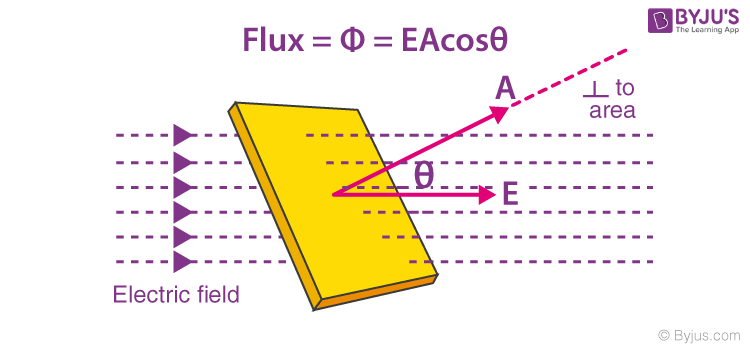# Flux of Electric Current

In Electromagnetism, the electric field is defined as the measure of the flow of electric current through a given area. It is a property of an electric field that may be thought as electric field lines that intersect at a particular area. These field lines arise from +ve electric charges and cut off at -ve charges. Electric flux is the rate at which the electric field flows through a given area. The electric flux is directly proportional to the number of electric field lines going through the virtual surface. Electric field lines that are conducted out of a sealed ( closed ) surface are +ve, and the lines that are carried into on a sealed surface are considered -ve.

Suppose if there is no net charge within a sealed surface than the field line that is conducted into the surface it continues to travel inwards of the surface and then it is led outwards somewhere on the surface. The net electric flux is ‘0’ when -ve flux is equal to +ve flux in magnitude. The unit of flux is Volt metres (V m)

Let E be the electric intensity at a point in an electric field. If the normal to the area A is along the direction of the electric field, then the flux through the small area A is given as

Φ = EA

If the normal to the area is at an angle θ to the direction of the field then the flux through the area A is given asΦ = EACos θ

Zero Flux: No electric field lines will pass through a surface when it placed parallel to the electric field.

Φ = EACos θ

= EA Cos 900

=  EA (0) = 0

Maximum Flux: The maximum electric field lines of force passes through a surface when it is placed perpendicular to the electric field.

Φ = EACos θ

= EA Cos 00

=  EA (1) = EA

## Gauss’s  Law

For an electric field, the mathematical relation between an enclosed charge and electric field is given by Gauss’s Law. It is one of the core law in electromagnetism. The Gauss law states that the total electric flux through a hypothetical closed surface is always equal to (1/ε0) times the net charge enclosed by the surface.

Φ = EACos θ = q/ε0

It simplifies the calculation for electric field for the geometry of sufficient symmetry. It is an important tool has it allows the appraisal of the volume of enclosed charges by mapping the field on a surface.

Test Your Knowledge On Flux Of Electric Current!